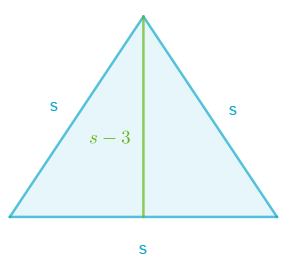### Math Notes

Subjects

#### Algebra Solutions

##### Topics || Problems

Find the length of a side of an equilateral triangle whose altitude is 3 feet shorter than a side.Solution 01:

Area of a triangle, $$A_T = \frac{1}{2} bh$$ and $$A_T = \frac{1}{2} s^2 \sin 60$$ for an equilateral triangle.

$$A_T = \frac{1}{2} bh$$

But $$b = s$$ and $$h = s+ 3$$

So, $$A_T = 0.5 s(s+3)$$

$$A_T = \frac{1}{2} s^2 \sin 60$$

$$A_T = \frac{\sqrt{3}}{4}s^2$$

Equate the two areas and simplify: $$\frac{\sqrt{3}}{4}s^2 = 0.5 s(s+3)$$

$$\frac{\sqrt{3}}{2}s = (s+3)$$

$$s (\frac{2-\sqrt{3}}{2}) = 3$$

$$s = 12 + 6\sqrt{3}$$

$$s = 22.39$$ ft

Solution 02:

Since the altitude forms a perpendicular line with the base, then new triangles are created. Since these triangles are right, use Pythagorean formula to calculate the side.

$$\sin 60 = \frac{s-3}{s}$$

$$s(\sin 60) = s-3$$

$$s(1-\sin 60) = 3$$

$$s = \frac{3}{1-\sin 60}$$

$$s = 12 + 6\sqrt{3}$$

$$s = 22.39$$ ft